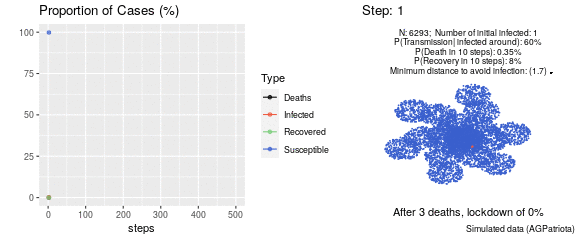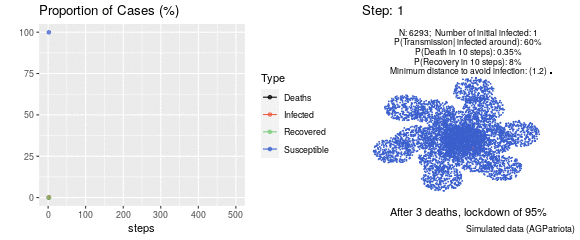University of São Paulo Institute of Mathematics and Statistics Alexandre Galvão Patriota Professor of Statistics (MS5) Department of Statistics IME - USP

Simulations in a (connected) clustered population.

In the simulations below, the parameters that lead to a strong change in the evolution of the curves seem to be the "minimum distance to avoid being infected" and the "probability of transmission if an infected trespasses the minimum distance to avoid infection". Nevertheless, the results are very sensible to the parameters.

Keep in mind that these are just simulations to understand how an epidemic evolves through the human net and they do not intend to represent reality.

I strongly encourage the reader to watch Simulating an epidemic by Grant Sanderson.

Watch the video: video

PS: The usual SIR model is not adequate to model the following cases. The parameters also vary with time.

Run the code by yourself Github. Forks with new scenarios would be very welcome. Let us share our knowledge!

First scenario:
No intervention
N = 6293
Number of initial infected = 1
Probability of transmission if an infected trespasses the minimum distance to avoid infection = 60%
Probability of an infected dying after 10 steps = 0.35%
Probability of an infected recover after 10 steps = 0.8%
Minimum distance to avoid infection = 0.8, 1.2, 1.7 and 2.2 (units of distance)
 1) Minimum distance to avoid infection from an infected 0.8. Should stay 0.8 (units) away from each other to avoid being infected (less aggressive).2)Same parameters as in 1) except: Minimum distance to avoid infection from an infected 1.2. Should stay 1.2 (units) away from each other to avoid being infected.3) Same parameters as in 1) except: (Minimum distance to avoid infection from an infected 1.7. Should stay 1.7 (units) away from each other to avoid being infected)4) Simulating an epidemic. Same parameters as in 1) except: Minimum distance to avoid infection from an infected 2.2. Should stay 2.2 (units) away from each other to avoid being infected (more aggressive)Second scenario:
The intervention: After 3 deaths, the lockdown applies for 95% of the population until the step 500 (they stay unmoved, but the other 5% can reach them).
N = 6293
Number of initial infected = 1
Probability of transmission if an infected trespasses the minimum distance to avoid infection = 60%
Probability of an infected dying after 10 steps = 0.35%
Probability of an infected recover after 10 steps = 0.8%
Minimum distance to avoid infection = 0.8, 1.2, 1.7 and 2.2 (units of distance)
 1)Minimum distance to avoid infection from an infected 0.8. Should stay 0.8 (units) away from each other to avoid being infected (less aggressive).2) Same parameters as in 1) except: Minimum distance to avoid infection from an infected 1.2. Should stay 1.2 (units) away from each other to avoid being infected.3) Same parameters as in 1) except: (Minimum distance to avoid infection from an infected 1.7. Should stay 1.7 (units) away from each other to avoid being infected)4) Same parameters as in 1) except: Minimum distance to avoid infection from an infected 2.2. Should stay 2.2 (units) away from each other to avoid being infected (more aggressive)